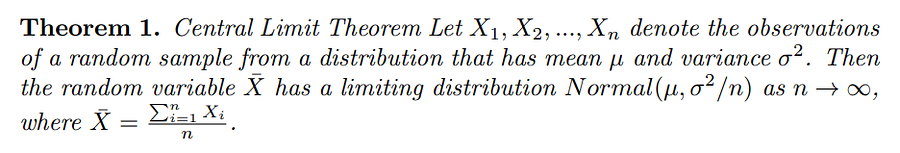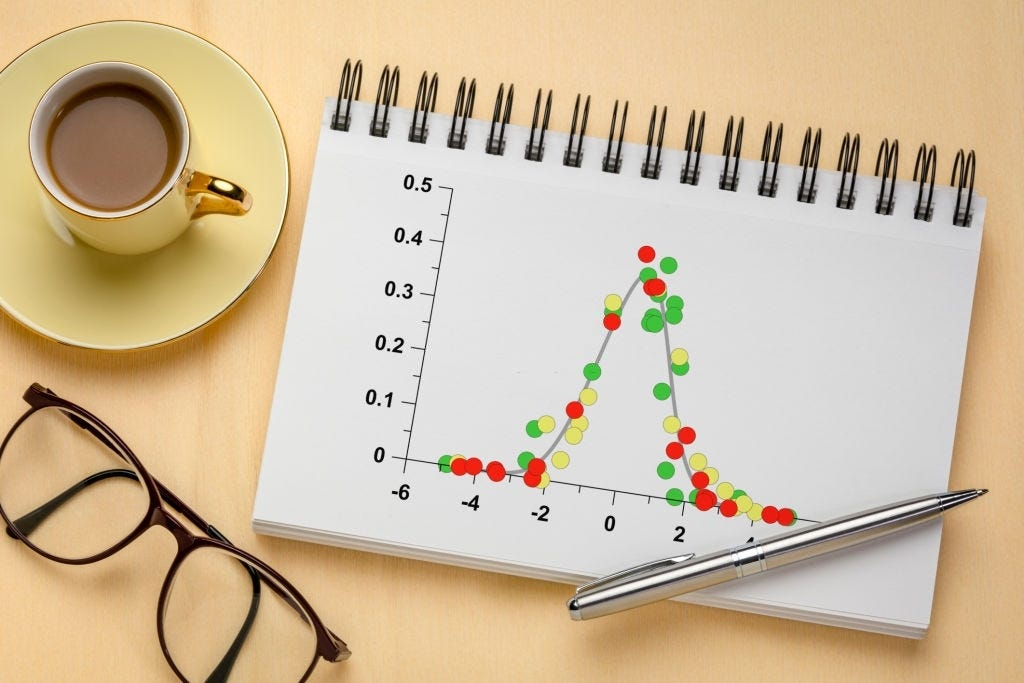1604157060

# Understanding the Central Limit Theorem

The central limit theorem (CLT) is a popular concept in statistics. I believe most (aspiring) data scientists have heard about it in some sort of form, or at least what the theorem is all about on a high level. The theorem is often said to magically offer interconnection between any data distribution to the normal (Gaussian) distribution when the data size is large.

With that being said, I observe the true concept of the theorem is rather unclear for many— including me. Yes, the theorem connects any distribution to the normal distribution. But what kind of connection? Unclear. And how is the process of achieving the connection? Also unclear.

Even worst for me, I once thought that the theorem implies any data will always follow the normal distribution when the size is large. Or in the other words, it is NOT possible to have skewed (or any non-normal) distribution on large data points. Crap!

Through this article, I will try to explain CLT so that you don’t get trapped in the same misunderstanding as mine in the above. In the end part, I also discuss the theorem’s importance in one of the core competencies that every data scientist should comprehend, namely hypothesis testing.

1. Revisiting the theorem
2. Debunking the key concept: “Sampling distribution of the mean”
3. Demo: a crazy dice
4. CLT in hypothesis testing

## The theorem

The following is the precise (mathematical) form of the central limit theorem (CLT).Don’t panic! As I don’t assume every one of you is a hardcore mathematician, I provide the “plain English” version of the theorem:

Given a sufficiently large sample size, the sampling distribution of the mean for a variable will apporximate a normal distribution regardless of that variable’s distribution in the population.

Even better, the mean of the sampling distribution of the mean is equal to the population mean.

Or on a higher level, the values of a variable can follow different probability distributions like below.

#central-limit-theorem #normal-distribution #hypothesis-testing #statistics #data-science